Next: Five Coplanar Points Up: Recognition with Invariants Previous: Recognition with Invariants

## Generalities

Four aligned points provide a projective invariant, the cross ratio. If we only allow affine transformations, three aligned points are enough to provide an invariant: the ratio of their separations. Finally, limiting ourselves to rigid planar motions, there is an invariant for only two points: their separation. In other words, invariants are only defined relative to a group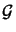of permissable transformations. The smaller the group, the more invariants there are. Here we will mainly concentrate on the group of planar projective transformations.

Invariants measure the properties of configurations that remain constant under arbitrary group transformations. A configuration may contain many invariants. For instance 4 points lead to 6 different cross ratios. However only one of these is functionally independent: once we know one we can trivially calculate the other five. In general, it is useful to restrict attention to functionally independant invariants.

An important theorem tells us how many independant invariants exist for a given algebraic configuration. Let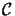be the configuration and dof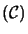its number of degrees of freedom, i.e. the number of independant parameters needed to describe it, or the dimension of the corresponding parameter manifold. Similarly, let dof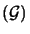be the number of independent parameters required to characterize a transformation of group; let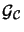be the subgroup ofthat leaves the configuration invariant as a shape; and let dof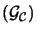be this isotropy subgroup's number of degrees of freedom. Then we have the following theorem :

Theorem: The number of independant invariants of a configurationunder transformationsis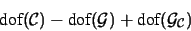Consider a few examples:

1.
Letbe the 2 dof group of affine transformations on the line. No continuous family of affine transformations leaves 3 collinear points (which have 3 dof) invariant: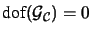. So there is 3-2+0=1 independant invariant, e.g. one of the length ratios.
2.
Letbe the 3 dof group of rigid transformations in the plane andbe the 3 dof configuration of two parallel lines.is the 1 dof subgroup of translations parallel to this line, so there is 3-3+1=1 independant invariant, e.g. the distance between the lines.
3.
Two conics in the projective plane have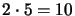dof. The planar projective group has 8 dof and no continuous family of projective transformations leaves two conics globally invariant; therefore there are 10-8+0=2 invariants for such a configuration.

In practice, the isotropy subgroupoften reduces to the identity. The assumption it has 0 dof is known as the counting argument''. However, it can be very difficult to spot isotropies, so care is needed:

Exercise 3.9   : In the projective plane, consider two lines L,L' and two points A, B in general position. How many invariants does the counting argument suggest? However there is at least one invariant: A,B and the intersections of AB with L and L' define a cross ratio. Exhibit the isotropy subgroup and show that it has 1 dof. (Hint: Map A, B to infinity and the intersection of the lines to the origin: what is the isotropy subgroup now?)Next: Five Coplanar Points Up: Recognition with Invariants Previous: Recognition with Invariants
Bill Triggs
1998-11-13